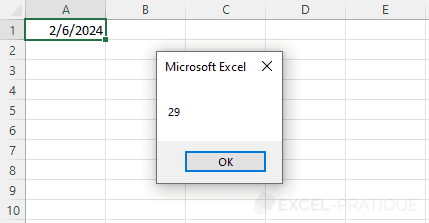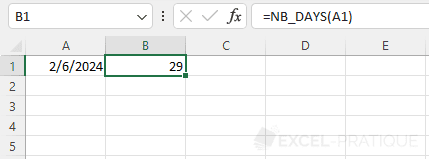# VBA Tip: How Many Days in a Month

You can use the following formula to calculate the number of days in a month or determine the last day in a month:

```Copy```Sub nb_days_month()

date_test = CDate("6/2/2012") 'Any date will do for this example

nb_days = Day(DateSerial(Year(date_test), Month(date_test) + 1, 1) - 1)

End Sub``````

Here is a version of the formula broken down into its constituent parts with commentaries that will help you understand how it works:

```Copy```Sub nb_days_month()

'Any date will do for this example
date_test = CDate("6/2/2012")

'Month / Year of the date
var_month = Month(date_test)
var_year = Year(date_test)

'Calculation for the first day of the following month
date_next_month = DateSerial(var_year, var_month + 1, 1)

'Date of the last day
last_day_month = date_next_month - 1

'Number for the last day of month (= last day)
nb_days = Day(last_day_month)

End Sub``````

## Function

You can also use the above code as a function by adding the following code to a module:

```Copy```Function NB_DAYS(date_test As Date)
NB_DAYS = Day(DateSerial(Year(date_test), Month(date_test) + 1, 1) - 1)
End Function``````

Example of how the function can be used in VBA:

```Copy```Sub example()
test = NB_DAYS(Range("A1"))
MsgBox test
End Sub``````Example of how the function can be used on a calculation worksheet: